# Number facts for kids

Kids Encyclopedia Facts
For the book in the Bible, see Numbers (Bible).

A number is a concept from mathematics, used to count or measure. Depending on the field of mathematics, where numbers are used, there are different definitions:

Numbers are also used for other things besides counting. Numbers are used when things are measured. Numbers are used to study how the world works. Mathematics is a way to use numbers to learn about the world and make things. The study of the rules of the natural world is called science. The work that uses numbers to make things is called engineering.

## Numbering methods

### Numbers for people

There are different ways of giving symbols to numbers. These methods are called number systems. The most common number system that people use is the base ten number system. The base ten number system is also called the decimal number system. The base ten number system is common because people have ten fingers and ten toes. There are 10 different symbols {0, 1, 2, 3, 4, 5, 6, 7, 8, and 9} used in the base ten number system. These ten symbols are called digits.

A symbol for a number is made up of these ten digits. The position of the digits shows how big the number is. For example, the number 23 in the decimal number system really means (2 times 10) plus 3, and 101 means 1 times a hundred (=100) plus 0 times 10 (=0) plus 1 times 1 (=1).

### Numbers for machines

Another number system is more common for machines. The machine number system is called the binary number system. The binary number system is also called the base two number system. There are two different symbols (0 and 1) used in the base two number system. These two symbols are called bits.

A symbol for a binary number is made up of these two bit symbols. The position of the bit symbols shows how big the number is. For example, the number 10 in the binary number system really means 1 times 2 plus 0, and 101 means 1 times four (=4) plus 0 times two (=0) plus 1 times 1 (=1). The binary number 10 is the same as the decimal number 2. The binary number 101 is the same as the decimal number 5.

## Names of numbers

See also: Names of numbers in English

English has special names for some of the numbers in the decimal number system that are "powers of ten". All of these power of ten numbers in the decimal number system use just the symbol "1" and the symbol "0". For example, ten tens is the same as ten times ten, or one hundred. In symbols, this is "10 × 10 = 100". Also, ten hundreds is the same as ten times one hundred, or one thousand. In symbols, this is "10 × 100 = 10 × 10 × 10 = 1000". Some other power of ten numbers also have special names:

When dealing with larger numbers than this, there are two different ways of naming the numbers in English. Under the "long scale" a new name is given every time the number is a million times larger than the last named number. It is also called the "British Standard". This scale used to be common in Britain but is not often used in English-speaking countries today. It is still used in some other European nations. Another scale is the "short scale" under which a new name is given every time a number is a thousand times larger than the last named number. This scale is a lot more common in most English-speaking nations today.

## Types of numbers

### Natural numbers

Natural numbers are the numbers which we normally use for counting, 1, 2, 3, 4, 5, 6, 7, 8, 9, 10 etc. Some people say that 0 is a natural number, too.

Another name for these numbers is positive numbers. These numbers are sometimes written as +1 to show that they are different from the negative numbers. But not all positive numbers are natural (for example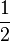$\frac{1}{2}$ is positive, but not natural).

If 0 is called a natural number, then the natural numbers are the same as the whole numbers. If 0 is not called a natural number, then the natural numbers are the same as the counting numbers. So if the words "natural numbers" are not used, then there will be less confusion about whether zero is included or not. But unfortunately, some say that zero is not a whole number, either, and some say whole numbers can be negative. "Positive integers" and "non-negative integers" are another way to include zero or exclude zero, but only if people know those words.

### Negative numbers

Negative numbers are numbers less than zero.

One way to think of negative numbers is using a number line. We call one point on this line zero. Then we will label (write the name of) every position on the line by how far to the right of the zero point it is, for example the point one is one centimeter to the right, the point two is two centimeters to the right.

Now think about a point which is one centimeter to the left of the zero point. We cannot call this point one, as there is already a point called one. We therefore call this point minus 1 (−1) (as it is one centimeter away, but in the opposite direction).

A drawing of a number line is below.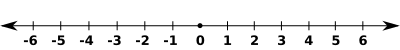All the normal operations of mathematics can be done with negative numbers:

If people add a negative number to another this is the same as taking away the positive number with the same numerals. For example, 5 + (−3) is the same as 5 − 3, and equals 2.

If they take away a negative number from another this is the same as adding the positive number with the same numerals. For example, 5 − (−3) is the same as 5 + 3, and equals 8.

If they multiply two negative numbers together they get a positive number. For example, −5 times −3 is 15.

If they multiply a negative number by a positive number, or multiply a positive number by a negative number, they get a negative result. For example, 5 times −3 is −15.

As finding the square root of a negative number is impossible as negative times negative equals possitve. We simbolise the square root of a negative number as i.

### Integers

Integers are all the natural numbers, all their opposites, and the number zero. Decimal numbers and fractions are not integers.

### Rational numbers

Rational numbers are numbers which can be written as fractions. This means that they can be written as a divided by b, where the numbers a and b are integers, and b is not equal to 0.

Some rational numbers, such as 1/10, need a finite number of digits after the decimal point to write them in decimal form. The number one tenth is written in decimal form as 0.1. Numbers written with a finite decimal form are rational. Some rational numbers, such as 1/11, need an infinite number of digits after the decimal point to write them in decimal form. There is a repeating pattern to the digits following the decimal point. The number one eleventh is written in decimal form as 0.0909090909 ... .

A percentage could be called a rational number, because a percentage like 7% can be written as the fraction 7/100. It can also be written as the decimal 0.07. Sometimes, a ratio is considered as a rational number.

### Irrational numbers

Irrational numbers are numbers which cannot be written as a fraction, but do not have imaginary parts (explained later).

Irrational numbers often occur in geometry. For instance if we have a square which has sides of 1 meter, the distance between opposite corners is the square root of two, which equals 1.414213 ... . This is an irrational number. Mathematicians have proved that the square root of every natural number is either an integer or an irrational number.

One well-known irrational number is pi. This is the circumference (distance around) of a circle divided by its diameter (distance across). This number is the same for every circle. The number pi is approximately 3.1415926535 ... .

An irrational number cannot be fully written down in decimal form. It would have an infinite number of digits after the decimal point. Unlike 0.333333 ..., these digits would not repeat forever.

### Real numbers

Real numbers is a name for all the sets of numbers listed above:

• The rational numbers, including integers
• The irrational numbers

This is all numbers that do not involve imaginary numbers.

### Imaginary numbers

Imaginary numbers are formed by real numbers multiplied by the number i. This number is the square root of minus one (−1).

There is no number in the real numbers which when squared makes the number −1. Therefore, mathematicians invented a number. They called this number i, or the imaginary unit.

Imaginary numbers operate under the same rules as real numbers:

• The sum of two imaginary numbers is found by pulling out (factoring out) the i. For example, 2i + 3i = (2 + 3)i = 5i.
• The difference of two imaginary numbers is found similarly. For example, 5i − 3i = (5 − 3)i = 2i.
• When multiplying two imaginary numbers, remember that i × i (i2) is −1. For example, 5i × 3i = ( 5 × 3 ) × ( i × i ) = 15 × (−1) = −15.

Imaginary numbers were called imaginary because when they were first found, many mathematicians did not think they existed.

### Complex numbers

Complex numbers are numbers which have two parts; a real part and an imaginary part. Every type of number written above is also a complex number.

Complex numbers are a more general form of numbers. The complex numbers can be drawn on a number plane. This is composed of a real number line, and an imaginary number line.

           3i|_
|
|
2i|_          . 2+2i
|
|
i|_
|
|
|_____|_____|_____|_____|_____|_____|_____|_____|
−2    −1     0     1     2     3     4     5     6
|
−i|_                .3−i
|
|
.−2−2i   −2i|_
|
|
−3i|_
|


All of normal mathematics can be done with complex numbers:

• To add two complex numbers, add the real and imaginary parts separately. For example, (2 + 3i) + (3 + 2i) = (2 + 3) + (3 + 2)i= 5 + 5i.
• To subtract one complex number from another, subtract the real and imaginary parts separately. For example, (7 + 5i) − (3 + 3i) = (7 − 3) + (5 − 3)i = 4 + 2i.

To multiply two complex numbers is complicated. It is easiest to describe in general terms, with two complex numbers a + bi and c + di.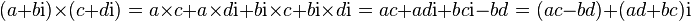$( a + b \mathrm{i} ) \times ( c + d\mathrm{i} ) = a \times c + a \times d\mathrm{i} + b\mathrm{i} \times c + b\mathrm{i} \times d\mathrm{i} = ac + ad\mathrm{i} + bc\mathrm{i} -bd = ( ac - bd ) + ( ad + bc )\mathrm{i}$

For example, (4 + 5i) × (3 + 2i) = (4 × 3 − 5 × 2) + (4 × 2 + 5 × 3)i = (12 − 10) + (8 + 15)i = 2 + 23i.

### Transcendental numbers

A real or complex number is called a transcendental number if it can not be obtained as a result of an algebraic equation with integer coefficients.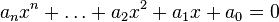$a_{n}x^{n} + \dots + a_{2}x^2 + a_{1}x + a_{0} = 0$

Proving that a certain number is transcendental can be extremely difficult. Each transcendental number is also an irrational number. The first people to see that there were transcendental numbers were Gottfried Wilhelm Leibniz and Leonhard Euler. The first to actually prove there were transcendental numbers was Joseph Liouville. He did this in 1844.

Well-known transcendental numbers:

• e
• π
• ea for algebraic a 0
•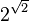$2^{\sqrt{2}}$

## Images for kidsNumber Facts for Kids. Kiddle Encyclopedia.